# Tanks Usage#

Tanks can be added to Hybrids and Liquids motors so that the propellant’s time varying properties can be properly calculated. To do this the tank must be first separately defined.

A few different types of tanks are available. These are simply different ways of defining a the propellant flow given different information. The different types of tanks are:

• `class MassFlowRateBasedTank`: flow is described by mass flow rate. See Mass Flow Rate Based Tank for more information.

• `class MassBasedTank`: flow is described by liquid and gas masses. See Mass Based Tank for more information.

• `class LevelBasedTank`: flow is described by liquid level. See Level Based Tank for more information.

• `class UllageBasedTank`: flow is described by ullage. See Ullage Based Tank for more information.

To summarize, the `UllageBasedTank` and `LevelBasedTank` are less accurate than the `MassFlowRateBasedTank` and `MassBasedTank`, since they assume uniform gas distribution filling all the portion that is not occupied by liquid. Therefore, these tanks can only model the tank state until the liquid runs out.

Tanks also must receive a `TankGeometry` object which describes the tank’s geometry. This object is defined in the Tank Geometry section.

Finally, tanks must be given a `Fluid` object which describes the propellant in the tank. This object is defined in the Fluid section.

Tanks are added to motors using the `add_tank` method of the motor. You can find more information about this method in the Adding Tanks section.

Attention

As always with rocketpy, the units of the density are in accordance with the International System of Units (SI).

## Fluid#

Fluid are a very simple class which describes the properties of a fluid. They are used to define the propellant in a tank. A Fluid is defined by its name and density as such:

```liquid_N2O = Fluid(name="Liquid Nitrous Oxide", density=855)
vapour_N2O = Fluid(name="Vapour Nitrous Oxide", density=101)
```

Fluid are then passed to tanks when they are defined.

## Tank Geometry#

When defining a tank, its geometry must be specified. The geometry can be defined in two ways:

• Using the predefined `CylindricalTank` and `SphericalTank` classes.

• Using the `TankGeometry` class to input a custom geometry.

### Cylindrical and Spherical Tanks#

The predefined `CylindricalTank` class is easy to use and is defined as such:

```cylindrical_geometry = CylindricalTank(radius=0.1, height=2.0, spherical_caps=False)
```

Note

The `spherical_caps` parameter is optional and defaults to `False`. If set to `True`, the tank will be defined as a cylinder with flat caps. If True, the tank will have spherical caps.

The predefined `SphericalTank` is defined with:

```spherical_geometry = SphericalTank(radius=0.1)
```

`rocketpy.CylindricalTank` and `rocketpy.SphericalTank` for more information on these parameters.

### Custom Tank Geometry#

The `TankGeometry` class can be used to define a custom geometry by passing the `geometry_dict` parameter, which is a dictionary with its keys as tuples containing the lower and upper bound of the tank, while the values correspond to the radius function of that section of the tank.

To exemplify, lets define a cylindrical tank with the same dimensions as the `CylindricalTank` example above:

```custom_geometry = TankGeometry(
geometry_dict={
(-1, 1): lambda x: 0.1,
}
)
```

This defines a cylindrical tank with a 2 m lengths (from -1 m to 1 m) and a constant radius of 0.1 m.

Note

The center of coordinate is always at the exact geometrical center of the tank.

We can also define a tank with a parabolic cross-section by using a variable radius, for example:

```custom_geometry = TankGeometry(
geometry_dict={
(-1, 1): lambda x: 0.1*x**2,
}
)
```

## Mass Flow Rate Based Tank#

A `MassFlowRateBasedTank` has its flow described by the variation of liquid and gas masses through time and is defined as such:

```N2O_flow_tank = MassFlowRateBasedTank(
name="MassFlowRateBasedTank",
geometry=cylindrical_geometry,
flux_time=24.750,
liquid=liquid_N2O,
gas=vapour_N2O,
initial_liquid_mass=42.8,
initial_gas_mass=0.1,
liquid_mass_flow_rate_in=0,
liquid_mass_flow_rate_out="../data/motors/liquid_motor_example/liquid_mass_flow_out.csv",
gas_mass_flow_rate_in=0,
gas_mass_flow_rate_out="../data/motors/liquid_motor_example/gas_mass_flow_out.csv",
discretize=100,
)
```

Important

Pay special attention to the `flux_time`, `liquid_mass_flow_rate_in`, `liquid_mass_flow_rate_out`, `gas_mass_flow_rate_in` and `gas_mass_flow_rate_out` parameters.

More details can be found in `rocketpy.MassFlowRateBasedTank.__init__`.

We can see some useful plots with:

```# Draw the tank
N2O_flow_tank.draw()
```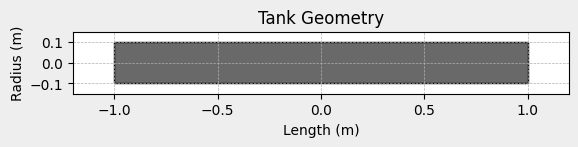```# Evolution of the Propellant Mass and the Mass flow rate
N2O_flow_tank.fluid_mass.plot()
N2O_flow_tank.net_mass_flow_rate.plot()
```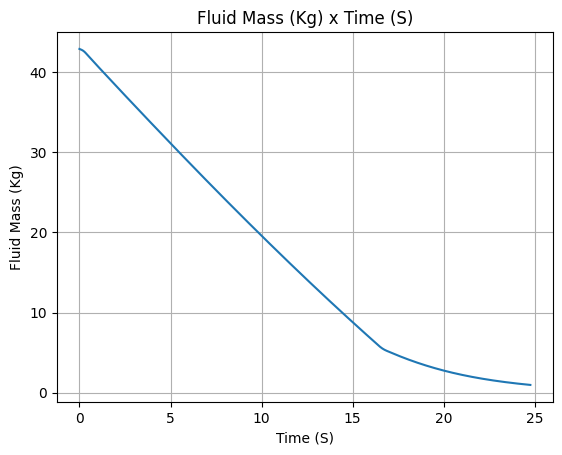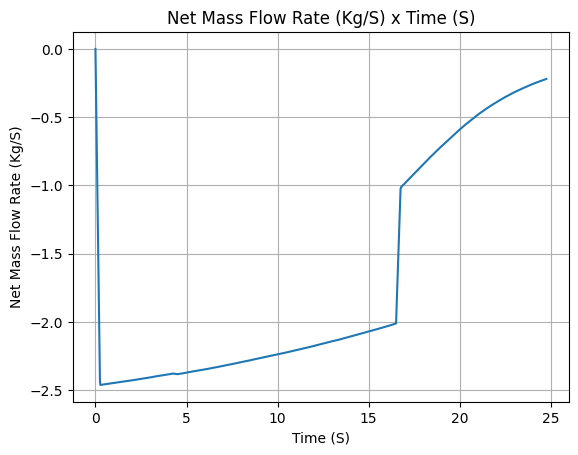```# Evolution of the Propellant center of mass position
N2O_flow_tank.center_of_mass.plot()
```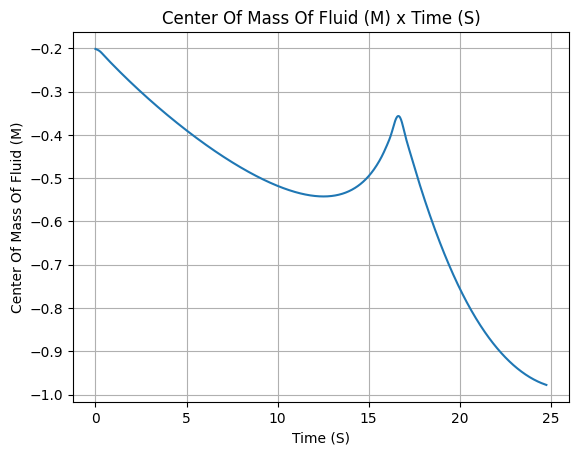## Mass Based Tank#

A `MassBasedTank` has its flow described by the variation of liquid and gas masses through time. To define it, lets get the liquid and gas masses from the `MassFlowRateBasedTank` we defined above:

```gas_mass = N2O_flow_tank.gas_mass
liquid_mass = N2O_flow_tank.liquid_mass
```

Then we can define the `MassBasedTank` as such:

```N2O_mass_tank = MassBasedTank(
name = "MassBasedTank",
geometry = cylindrical_geometry,
flux_time = 24.750,
gas = vapour_N2O,
liquid = liquid_N2O,
gas_mass = gas_mass,
liquid_mass = liquid_mass,
discretize=100,
)
```

Important

Pay special attention to the `flux_time`, `gas_mass` and `liquid_mass` parameters.

More details can be found in `rocketpy.MassBasedTank.__init__`.

We can see some outputs with:

```# Draw the tank
N2O_mass_tank.draw()
``````# Evolution of the Propellant Mass and the Mass flow rate
N2O_mass_tank.fluid_mass.plot()
N2O_mass_tank.net_mass_flow_rate.plot()
```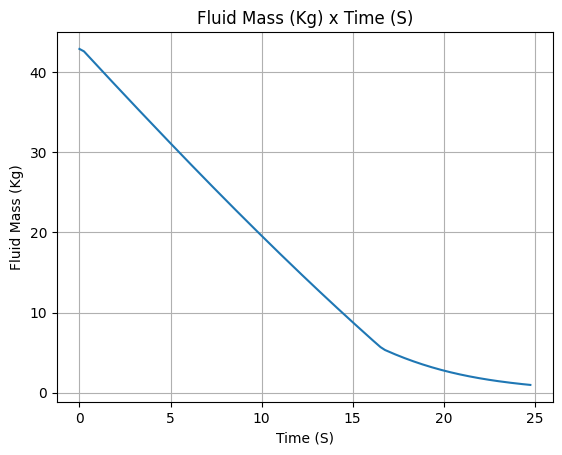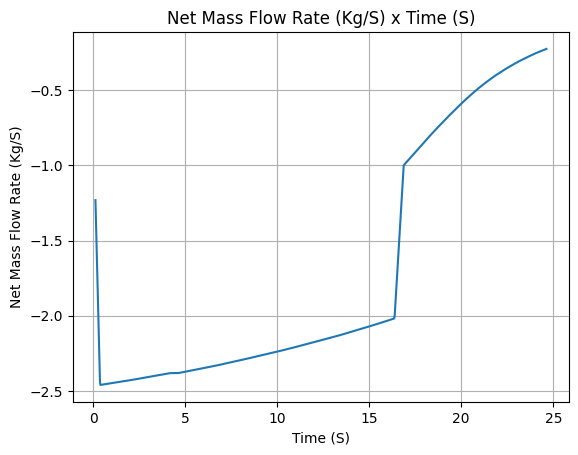```# Evolution of the Propellant center of mass position
N2O_mass_tank.center_of_mass.plot()
```## Ullage Based Tank#

An `UllageBasedTank` has its flow described by the ullage volume, i.e., the volume of the tank that is not occupied by the liquid. It assumes that the ullage volume is uniformly filled by the gas.

To define it, lets first calculate the ullage volume by using the `MassFlowRateBasedTank` we defined above:

```tank_volume = cylindrical_geometry.total_volume
ullage = (-1 * N2O_flow_tank.liquid_volume) + tank_volume
```

Then we can define the `UllageBasedTank` as such:

```N2O_ullage_tank = UllageBasedTank(
name="UllageBasedTank",
geometry=cylindrical_geometry,
flux_time=24.750,
gas=vapour_N2O,
liquid=liquid_N2O,
ullage=ullage,
discretize=100,
)
```

Important

Pay special attention to the `flux_time` and `ullage` parameters.

More details can be found in `rocketpy.UllageBasedTank.__init__`.

We can see some outputs with:

```# Draw the tank
N2O_ullage_tank.draw()
``````# Evolution of the Propellant Mass and the Mass flow rate
N2O_ullage_tank.fluid_mass.plot()
N2O_ullage_tank.net_mass_flow_rate.plot()
``````# Evolution of the Propellant center of mass position
N2O_ullage_tank.center_of_mass.plot()
```## Level Based Tank#

A `LevelBasedTank` has its flow described by liquid level, i.e., the height of the liquid inside the tank. It assumes that the volume above the liquid level is uniformly occupied by gas.

To define it, lets first calculate the liquid height by using the `MassFlowRateBasedTank` we defined above:

```liquid_height = N2O_flow_tank.liquid_height
```

Then we can define the `LevelBasedTank` as such:

```N20_level_tank = LevelBasedTank(
name="LevelBasedTank",
geometry=cylindrical_geometry,
flux_time=24.750,
liquid=liquid_N2O,
gas=vapour_N2O,
liquid_height=liquid_height,
discretize=100,
)
```

Important

Pay special attention to the `flux_time` and `liquid_height` parameters.

More details can be found in `rocketpy.LevelBasedTank.__init__`.

We can see some outputs with:

```# Draw the tank
N20_level_tank.draw()
```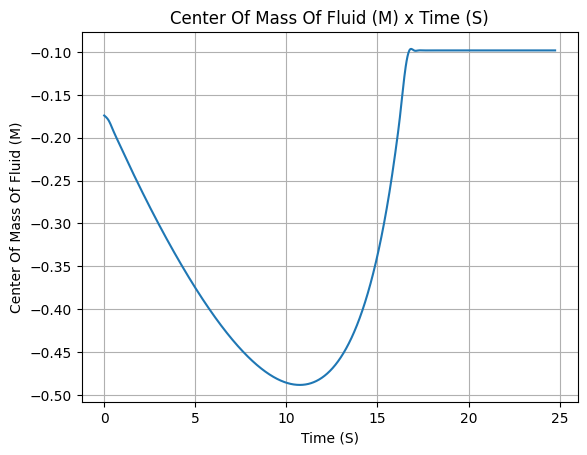```# Evolution of the Propellant Mass and the Mass flow rate
N20_level_tank.fluid_mass.plot()
N20_level_tank.net_mass_flow_rate.plot()
``````# Evolution of the Propellant center of mass position
N20_level_tank.center_of_mass.plot()
```## Comparing Tanks#

Now that we saw the different methods to calculate the mass flow rate, we can compare the results all together.

```tanks = [N2O_flow_tank, N2O_ullage_tank, N2O_mass_tank, N20_level_tank]
```
```# Mass
Function.compare_plots(
plot_list=[(tank.fluid_mass, tank.name) for tank in tanks],
lower=0,
upper=24.750,
title="Mass of Propellant in the Tank",
xlabel="Time (s)",
ylabel="Mass (kg)",
)
```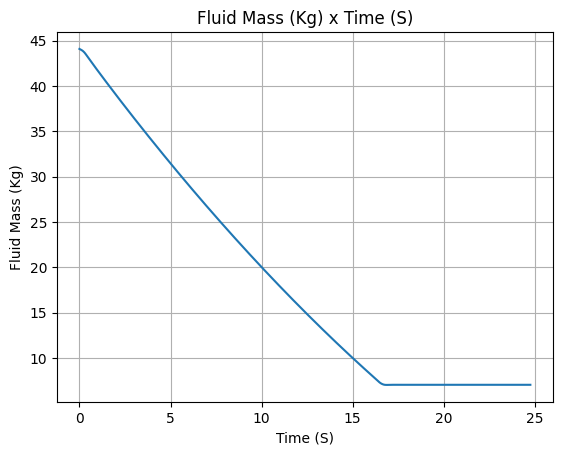```# Mass flow rate
Function.compare_plots(
plot_list=[(tank.net_mass_flow_rate, tank.name) for tank in tanks],
lower=0,
upper=24.750,
title="Mass Flow Rate Comparison",
xlabel="Time (s)",
ylabel="Mass Flow Rate (kg/s)",
)
```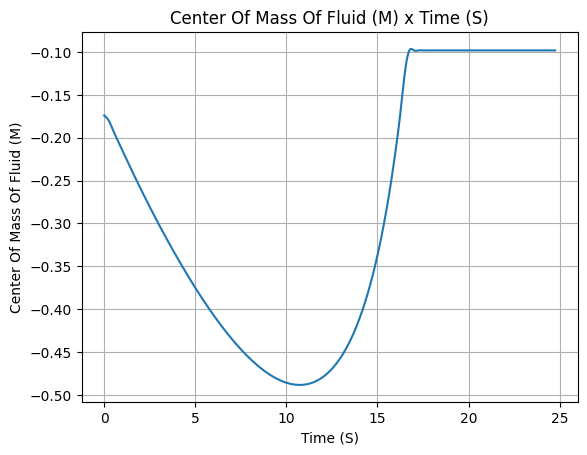```# Center of mass
Function.compare_plots(
plot_list=[(tank.center_of_mass, tank.name) for tank in tanks],
lower=0,
upper=24.750,
title="Center of Mass Comparison",
xlabel="Time (s)",
ylabel="Center of mass of Fluid (m)",
)
```